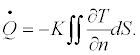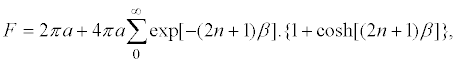Reach Us+44-1522-440391
Shape Factor for Steady State Heat Transfer between Swimming Pool Water and Surrounding Ground | OMICS International
Journal of Fundamentals of Renewable Energy and Applications
All submissions of the EM system will be redirected to Online Manuscript Submission System. Authors are requested to submit articles directly to Online Manuscript Submission System of respective journal.

# Shape Factor for Steady State Heat Transfer between Swimming Pool Water and Surrounding Ground

Aneesh Somwanshi1*, Amrit Dixit1 and Anil Kumar Tiwari2

1Disha Institute of Management and Technology, Raipur 492101, India

2National Institute of Technology, Raipur 492001, India

Corresponding Author:
Aneesh Somwanshi
Disha Institute of Management and Technology
Raipur 492101, India
Tel: +919752322262
E-mail: [email protected]

Received date: December 20, 2013; Accepted date: January 29, 2014; Published date: February 06, 2014

Citation: Somwanshi A, Dixit A, Anil Kumar T (2013) Shape Factor for Steady State Heat Transfer between Swimming Pool Water and Surrounding Ground. J Fundam Renewable Energy Appl 4:128 doi:10.4172/2090-4541.1000128

Copyright: © 2013 Somwanshi A, et al. This is an open-access article distributed under the terms of the Creative Commons Attribution License, which permits unrestricted use, distribution, and reproduction in any medium, provided the original author and source are credited.

Visit for more related articles at Journal of Fundamentals of Renewable Energy and Applications

#### Abstract

A simple, efficient, and abundantly available agricultural waste material, banana pseudo-stem (BPS) was examined as raw material for the extraction of potassium. The effects of various process parameters such as temperature, initial pH, contact time, banana pseudo-stem dosage and size of banana pseudo-stem particles on potassium extraction efficiency were studied by running batch experiments in Erlenmeyer flasks. Response Surface Methodology (RSM) was used to design the experimental runs. Modelling and optimization of process variables to obtain maximum extraction of potassium from raw material were done using RSM.The maximum extraction efficiency of potassium was found to be 83.96% at a temperature of 400C, pH of 1, contact time of 30 min, BPS weight of 26.076 g and initial BPS size of 300. The results revealed that banana pseudo-stem can be used as good source for potassium extraction.

#### Keywords

Shape factor, Thermal simulation, Characteristic length, Swimming pool

#### Introduction

Solar energy has been widely used for heating of swimming pools; a number of papers [1-13] on the implementation, analysis and validation, corresponding to the exploitation of various options have been published. The starting point for all the analyses is an energy balance equation, which takes into account the relevant modes of energy exchange between the pool and the surroundings; however a significant aspect viz. the energy exchange between the pool water and the surrounding ground has either been ignored or inadequately addressed to (usually based on one dimensional flow of heat in the ground). In this paper the authors have used the method of experimental simulation for the evaluation of the energy exchange between the pool water and the surrounding ground in the steady state. Such an investigation is also relevant to temperature conditioning of water in a pool by exchange of heat with earth, as proposed recently . The energy storage in the ground has for long been exploited for heating and cooling of buildings [15-19] and hence some attention (not enough) has been given to the study of energy exchange between a building and surrounding ground. For a theoretical analysis one has to solve the Fourier equation of heat conduction with appropriate boundary conditions; except for the simplest cases with high symmetry [20-22] it is not possible to obtain analytical solutions. Numerical solutions have of course been obtained [23-26] for structures with less symmetry. Full scale experiments, to evaluate the rate of heat exchange between a building and surrounding ground are not practicable; further the results are of little use on account of the variety of building designs.

With this background Sodha et al. [27-30] have developed the methods of electrical and thermal simulation (by experiment) to evaluate the heat transfer between a fully or partially underground structure and surrounding ground, where by results on small scale model can be scaled up for bigger realistic situations.

#### Theoretical Basis

Sodha et al. [27,10,31] modeled the steady state temperature distribution in the ground, the boundary conditions and the heat exchange between the body and the ground viz,

2(T) = 0,  (1a)

T (z=0) = Tg,  (1b)

T(Surface of body) =Ts  (1c)

and,(1d)

The method of simulation is based on the fact [27,31] that in general for the steady state Eq. (1d) may be put in the form,

Q = KLF(Ts −Tg ),  (2)

Where F depends on the relative (divided by L) dimensions of the body, relative depth and the orientation of the body and L is a characteristic dimension. Thus the steady state heat transfer between a body and the ground under any situation (characterized by L, thermal conductivity K of the medium and (Ts-Tg)) can be evaluated with a knowledge of the shape factor F, which may be determined by an experiment on a convenient scale or value of L (keeping the relative depth, relative dimensions of the body and the orientation of the body with respect to the surface the same) and convenient values of K and (Ts-Tg). In this paper, the shape factor corresponding to the heat transfer between the ground and three designs of swimming pools have been obtained by simulated experiments on a small scale.

#### Experiment

This paper reports on the experimental determination of the shape factor for heat transfer between the pool water and the ground for the following three designs:-

(I) Length (l) = 60m, Breadth (b) = 25m, Depth (d) = 2m. (Olympic size horizontal base pool ).

(II) Length (l) = 60m, Breadth (b) = 30m: vertical section normal to the breadth is shown in Figure (1a). (Slanted base pool ).

(III) Length (l) = 35ft, Breadth (b) = 20ft: vertical section normal to the breadth is shown in Figure (1b). (Diving pool ).

In all the cases the characteristic length is L= b/2 and the pool is full of water; the water surface is on level with the horizontal ground surface. The models are open boxes without cover; for the three pool designs the simulated dimensions are reduced by the reduction factor as follows:-

(I) Design I= (1/400). (II) Design II= (1/300). (III) Design III= (1/150).

The corresponding characteristic length L= b/2 for these models are 3.125×10-2 m, 5×10-2 m and 2×10-2 m, respectively. The models are closed by thick insulating pads at the top face.

Determination of Thermal conductivity of sand

The ground is simulated by a 1.5 m×l.5 m×l.5 m box full of sand. The thermal conductivity of the sand is determined before every experiment for determining the shape factor. The experimental arrangement for determination of the thermal conductivity of sand is diagrammatically illustrated in Figure 2. A hollow copper sphere of diameter (2a) 6 cm is buried in the sand, so that the Centre of the sphere is at depth d. There is an incandescent bulb or an insulated heating element in the sphere which is connected to a source of alternating emf. The rms values of the potential difference (V) and the current (I) flowing through the bulb/ element is measured; in the steady state the power output of the bulb/ element is equal to the power loss from sphere to the ground. Hence from Eq. (2) one gets,

Figure 2: Experimental set up to measure thermal conductivity of sand. 1. Sphere with mica insulation heating element. 2. Voltmeter 3. Microammeter. 4. Temperature sensor. 5. Temperature sensor. 6. Rheostat.

VI = FaK(Ts-Tg);  (3)

Here the radius a of the sphere is the characteristic length. The temperature difference (Ts-Tg) is determined by thermocouples. The shape factor F for a sphere of radius a at a depth d in the ground has been analytically evaluated [29,34] as,(4)

Where,

coshβ=(d/a)

Substituting for F from Eq.(4) in Eq. (3) and using the measured steady state values of V, I, Ts,Tg and a, the thermal conductivity of sand can be determined for d/a= 2 and 3, It is seen from Eq.(4) that the values of shape factor F are 15.3 and 14.5 for d/a= 2 and 3. From three readings for d/a= 2 and three readings for d/a= 3 on different days the authors obtained the value of thermal conductivity of sand K=0.271 ± 0.004W/m°C. A value of K=0.271W/m°C has been used in the evaluation of shape factors, corresponding to the three pool designs.

Determination of shape factor F for three pool designs

The experimental arrangement is diagrammatically illustrated in Figure 3. The copper model of the pool is so buried in sand that the top horizontal surface of the sand is in level with the open face of the model. Ceramic insulation is placed on the model, so that there is no heat loss from the top side. A heating element with mica insulation is placed inside the model and connected to an A.C source, through a mille ammeter and rheostat; the potential difference across the element and the current passing through it are measured by a voltmeter and micro ammeter. Temperature sensors measure the temperature difference (Ts-Tg) between the surface of the model and the top surface of the sand. Observations are taken when steady state values of Ts and Tgare indicated.

Figure 3: Experimental set up to find shape factor of the three pool designs. 1. Reduced scale pool model with heater element 2. Voltmeter. 3. Microammeter. 4. Temperature sensor. 5. Temperature sensor. 6. Variac. 7. Ceramic insulation. 8. Mica insulated heating element.

Referring to the half width of the pool as the characteristic length Eq. (2) may be rewritten as

VI = F (b/2) K (Ts-Tg);  (5)

V and I are the r.m.s values of the potential difference across and the current through the heating element. Knowing all the terms in this equation the shape factor F for the specific pool designs can be evaluated. It may be remembered that as stated earlier in the paper thermal conductivity K for sand is taken as 0.271W/m°C.

#### Observations and Results

These are presented in Tables 1-3. Thus the shape factors for designs I, II, and III are (16.77 ± 0.73), (15.91 ± 0.15) and (34.12 ± 1.38) the uncertainty in the values is less than 5% and should be acceptable for design purpose.

#### Conclusion

Experimental simulation has been used to evaluate the shape factor F, corresponding to steady state heat transfer between water in a swimming pool and the surrounding ground; the shape factor has been determined, corresponding to three designs of the swimming pool. The uncertainty in the values of F is less than 5% and thus acceptable for design purpose.

#### Acknowledgements

The authors are grateful to Prof. MS Sodha, Visiting and Emeritus Professor, CES, IIT Delhi, India for suggesting the problem and valuable guidance.

#### References

Select your language of interest to view the total content in your interested language

### Article Usage

• Total views: 12574
• [From(publication date):
August-2014 - Jul 18, 2019]
• Breakdown by view type
• HTML page views : 8697Can't read the image? click here to refresh